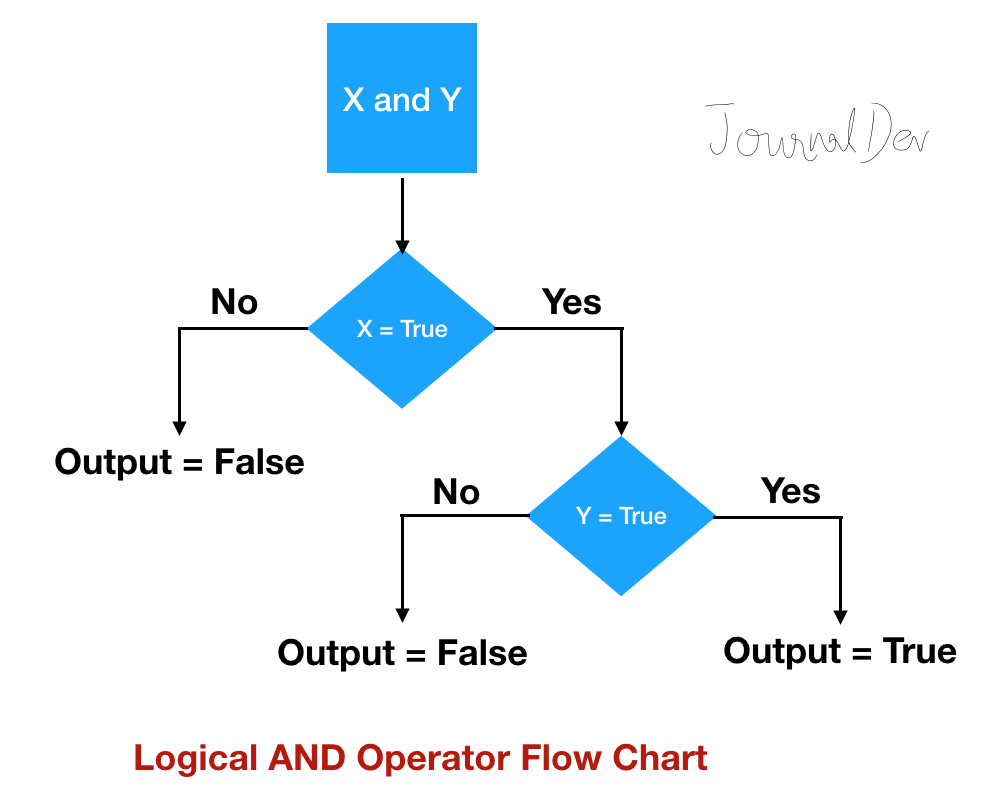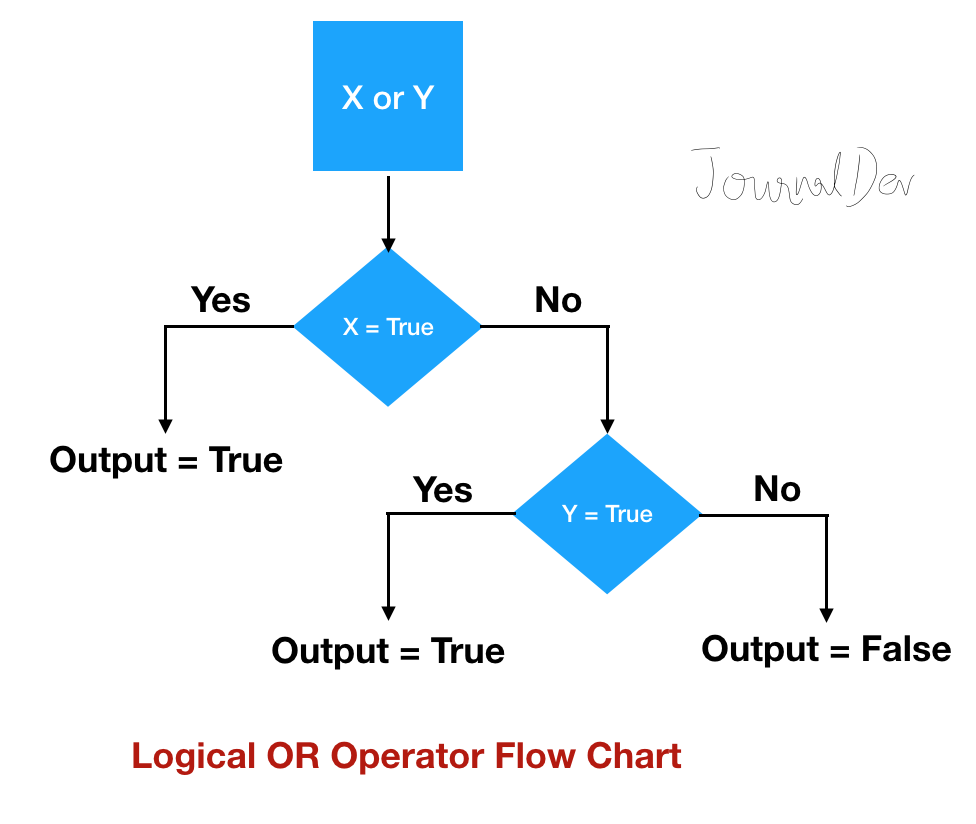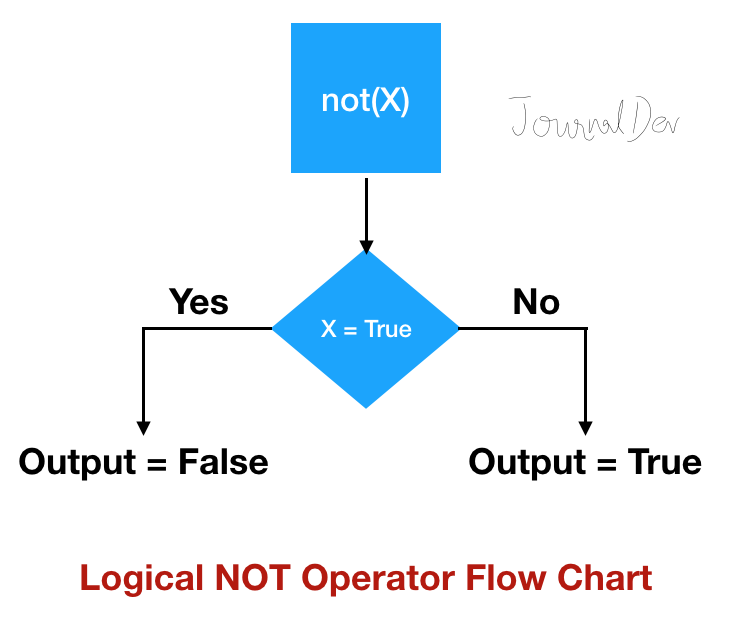### python 逻辑运算符_Python逻辑运算符

python 逻辑运算符

Python logical operators allow us to perform logical AND, OR, and NOT operation between boolean values. In this article, we will look into the logical operator’s execution in more detail.

Python逻辑运算符使我们能够在布尔值之间执行逻辑AND，OR和NOT运算。 在本文中，我们将更详细地研究逻辑运算符的执行。

## Python逻辑运算符 (Python Logical Operators)

There are three logical operators in Python.

Python中有三个逻辑运算符。

Logical Operator Description Simple Example
and Logical AND Operator flag = True and True = True
or Logical OR Operator flag = False or True = True
not Logical NOT Operator flag = not(False) = True

## Python逻辑AND运算子 (Python Logical AND Operator)

Here is a simple example of a logical and operator.

``x = 10y = 20 if x > 0 and y > 0:    print('Both x and y are positive numbers')``

Output: `Both x and y are positive numbers`

Before the logical operation is performed, the expressions are evaluated. So the above if condition is similar to below:

``if (x > 0) and (y > 0):    print('Both x and y are positive numbers')``

In Python, every variable or object has a boolean value. We have explained this in more detail at Python bool() function.

``if x and y:    print('Both x and y have boolean value as True')``

Output: `Both x and y have boolean value as True` because ‘x’ and ‘y’ len() function will return 2 i.e. > 0.

`False`, then the further expressions are not evaluated.
`False` ，则不对其他表达式进行计算。

Let’s confirm the above statement by defining a custom object. Python uses `__bool__()` function to get the boolean value of an object.

``class Data:    id = 0     def __init__(self, i):        self.id = i     def __bool__(self):        print('Data bool method called')        return True if self.id > 0 else False  d1 = Data(-10)d2 = Data(10)if d1 and d2:    print('Both d1 and d2 ids are positive')else:    print('Both d1 and d2 ids are not positive')``

Output:

``Data bool method calledAt least one of d1 and d2 ids is negative``

Notice that __bool__() function is called only once, confirmed from the print statement output.

Below diagram depicts the flow chart of logical and operation.Python Logical and Operator Flow Chart

Python逻辑和运算符流程图

Python ternary operator.
Python三元运算符 。

## Python逻辑或运算符 (Python Logical OR Operator)

Let’s look at a simple example of logical OR operator.

``x = 10y = 20 if x > 0 or y > 0:    print('At least one of x and y is positive number')``

Output: `At least one of x and y is positive number`

`True`, then the further expressions are not evaluated.
`True` ，则不对其他表达式进行计算。

Let’s confirm this with a simple code snippet. We will reuse the Data class defined earlier.

``d1 = Data(10)d2 = Data(20) # The expressions are evaluated until it's requiredif d1 or d2:    print('At least one of d1 and d2 id is a positive number')else:    print('Both d1 and d2 id are negative')``

Output:

``Data bool method calledAt least one of d1 and d2 id is a positive number``

Notice that the __bool__() method is called only once and since it’s evaluated to `True`, further expressions are not evaluated.

Let’s run another example where both Data object boolean value will return `False`.

``d1 = Data(-10)d2 = Data(-20) if d1 or d2:    print('At least one of d1 and d2 id is a positive number')else:    print('Both d1 and d2 id are negative')``

Output:

``Data bool method calledData bool method calledBoth d1 and d2 id are negative``

Finally, below image depicts the flow chart of logical or operator.Python Logical or Operator

Python逻辑或运算符

## Python逻辑非运算符 (Python Logical NOT Operator)

This is a very simple operator that works with a single boolean value. If the boolean value is `True` then not operator will return `False` and vice-versa.

Let’s look at a simple example of “not” operator.

``a = 37if not (a % 3 == 0 or a % 4 == 0):    print('37 is not divisible by either 3 or 4')``

Output: `37 is not divisible by either 3 or 4`Python Logical Not Operator

Python逻辑非运算符

## 从左到右评估Python运算符 (Python Operators Are Evaluated From Left to Right)

Let’s look at an example where we have multiple logical operators. We are not mixing different types of Python operators, so that operator precedence won’t come in picture and it will be easier to explain the output.

We will also override the __bool__() function to print “id” value.

``def my_bool(data):    print('Data bool method called, id =', data.id)    return True if data.id > 0 else False  Data.__bool__ = my_bool d1 = Data(10)d2 = Data(-20)d3 = Data(20) # evaluated from left to right, confirmed from the __bool__() print statementif d1 and d2 or d3:    print('At least one of them have a positive id.')``

Output:

``Data bool method called, id = 10Data bool method called, id = -20Data bool method called, id = 20At least one of them have a positive id.``

Notice that the boolean value is calculated for d1 first, thus confirming that the execution is happening from left to right.

## 逻辑运算符可用于任何自定义对象吗？ (Logical operators work with any custom object?)

In Python, every object has a boolean value. So logical operators will work with any custom objects too. However, we can implement __bool__() function to define our custom rules for an object boolean value.

## 摘要 (Summary)

Python logical operators allow us to perform logical operations. We looked into logical operators in detail, how their execution is optimized to take the least amount of time. We also confirmed that the operator’s execution is performed from left to right side.

Python逻辑运算符使我们能够执行逻辑运算。 我们详细研究了逻辑运算符，如何优化它们的执行以花费最少的时间。 我们还确认操作员的执行是从左到右执行的。

GitHub Repository.
GitHub存储库中检出完整的python脚本和更多Python示例。

python 逻辑运算符